Electronics

# Wheatstone bridge formula Class 12

The Wheatstone bridge circuit is widely used to precisely measure resistance. Also, the bridge is used in conjunction with transducers to measure physical quantities such as strain, temperature, and pressure. Transducers are devices that sense a change in a physical parameter and convert that change into an electrical quantity such as a change in resistance. Wheatstone bridge formula is provided here also.

For example, a strain gauge exhibits a change in resistance when it is exposed to mechanical factors such as force, pressure, or displacement. A thermistor exhibits a change in its resistance when it is exposed to a change in temperature. The Wheatstone bridge can be operated in a balanced or an unbalanced condition. The condition of operation depends on the type of application.

## What is Wheatstone bridge?

A Wheatstone bridge circuit is shown in its most common “diamond” configuration. It consists of four resistors, a de voltage source connected across the top and bottom point of the “diamond”. The output voltage is taken across the left and right points of the “diamond” between A and B. In part (b), the circuit is drawn in a slightly different to more clearly show its series-parallel configuration.

### Wheatstone Bridge formula

The Wheatstone bridge is in the balanced bridge condition when the output voltage (V OUT) between terminals A and B is equal to zero.

OUT  = 0 V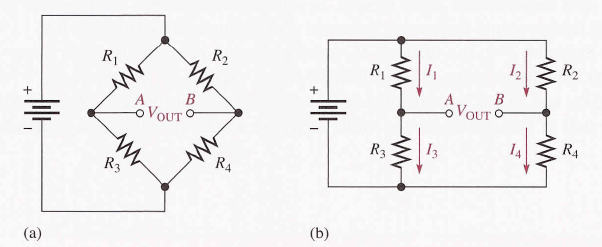When the bridge is balanced, the voltages across R1 and Rare equal (V= V ) and the voltages across Rand R4 are equal (V= V). Therefore, the voltage ratios can be written as: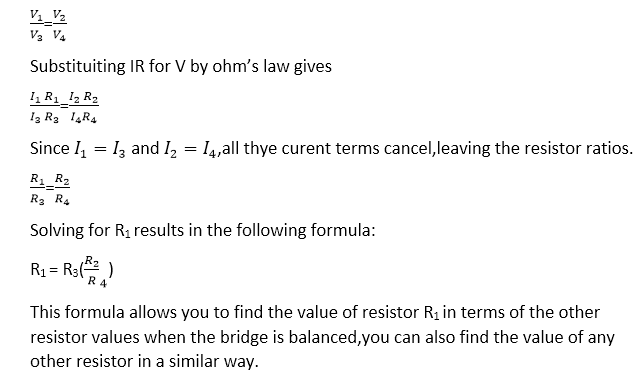### Using the Balance Wheatstone Bridge to Find unknown Resistance

Assume that R1 has an unknown value, which we call Rx. Resistor Rand R4 have fixed values so their ratio,R2/ R, also has a fixed value. Since Rcan be any value, R3 must be adjusted to make R2/ R = R/ Rin order to create a balanced condition. Therefore, Ris a variable resistor, which we will call Rx. When Ris placed in the bridge, Ris adjusted until the bridge is balanced as indicated by a zero output voltage. Then, the unknown resistance is found as: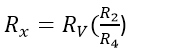The ratio R2/ Ris the scale factor.

Commonly, a measuring instrument called a galvanometer is connected between the output terminals A and B is detect a balanced condition. The galvanometer is essentially a very sensitive ammeter that senses current in either direction. It differs from a regular ammeter in that the mid-scale point is zero. So if the current through it is one direction, the needle deflects toward the positive side. If the current is in one direction, the needle deflects toward the negative side. When the needle is at the midpoint, the bridge is balanced because the voltage from A to B is zero and the current the galvanometer is zero, as illustrated. A voltmeter can also be used to indicate when the bridge is balanced.

From equationthe value of Rthe balance multiplied by the scale factor R/Ris the actual resistance value of R.If R/R4 = 1,then R= Rv, if R2 / R= 0.5, then R= 0.5 R, and so on. In a practical bridge circuit, the positive of the Radjustment can be calibrated to indicate the actual value of Ron a scale or with some other method of display.

### The Unbalanced Wheatstone Bridge

The unbalanced bridge, when VOUT is not equal to zero, is used to measure several types of physical quantities such as mechanical strain, temperature, or pressure. This can be done by connecting a transducer in one leg of the bridge. The resistance of the transducer changes proportionally to the changes in the parameter that it is measuring. If the bridge is balanced at a known point, then the amount of deviation from the balanced condition, as indicated by the output voltage, indicates the amount of change in the parameter being measured. Therefore, the value of the parameter being measured can be determined by the amount that the bridge is unbalanced.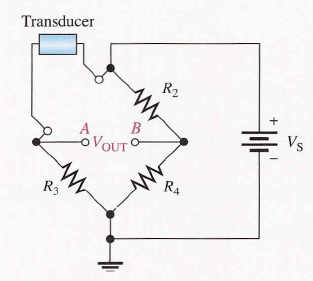### A Bridge Circuit For Measuring Temperature:

If the temperature to be measured, the transducer can be a thermistor, which is a temperature-sensitive resistor. The thermistor resistance change in a predictable way as the temperature changes. A change in temperature causes a change in thermistor resistance, which causes a corresponding change in the output voltage of the bridge as it becomes unbalanced. The output voltage is proportional to the temperature; therefore, either a voltmeter connected across the output can be calibrated to show the temperature or the output voltage can be amplified and converted to digital form to drive a readout display of the temperature.

A bridge circuit used to measure temperature is designed so that it is balanced at a reference temperature and becomes unbalanced at a measured temperature. For example, let’s say the bridge is to be balanced at 25°C. A thermistor will have a known value of resistance at 25°C. For simplicity, let’s assume the other three bridge resistors are equal to the thermistor resistance at 25 °C, so Rtherm  = R2 = R= R. For this particular case, the change in output voltage (ΔV OUT ) can be shown to be related to the change in Rtherm by the following formula.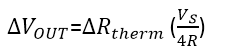The Δ (Greek letter delta) in front of a variable means a change in the variable. This formula applies only the case where all resistances in the bridge are equal when the bridge is balanced. Keep in mind that the bridge can be initially unbalanced without having all the resistors equal as long R= Rand R = R, but the formula for ΔV OUT would be more complicated.

## Wheatstone bridge examples

Determine the output voltage of the temperature-measuring bridge circuit. If the thermistor is exposed to a temperature of 50°C and its resistance at 25°C is 1.0 kΩ. Assume the resistance of the thermistor decreases to 900 Ω at 50° C.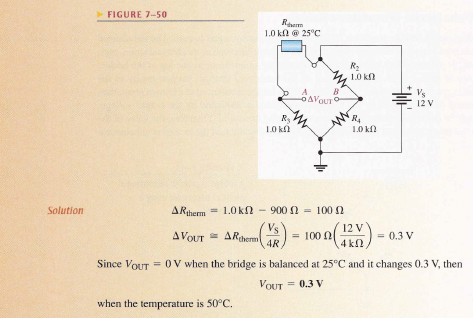## Wheatstone bridge uses

A Wheatstone bridge with a strain gauge can be used to measure certain forces. A strain gauge is a device that exhibits a change in resistance when it is compressed or stretched by the application of an external force. As the resistance of the strain gauge changes, the previously balanced bridge becomes unbalanced.This unbalance causes the output voltage to change from zero, and this change can be measured to determine the amount of strain. In strain gauges, the resistance change is extremely small. This tiny change unbalances a Wheatstone bridge because of its high sensitivity. For example, a Wheatstone bridge with strain gauges is commonly used in precision weight scales.

A Wheatstone bridge can be used in a similar way for measuring pressure using a pressure transducer. The application of pressure changes the resistance of the transducer, causing the bridge to become unbalanced by an amount proportional to the pressure.

## Wheatstone bridge animation

Related Topics:

1.Alla says: